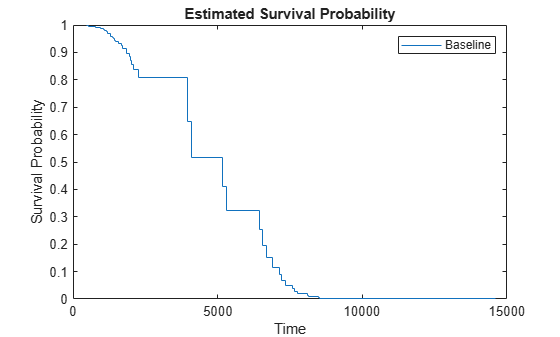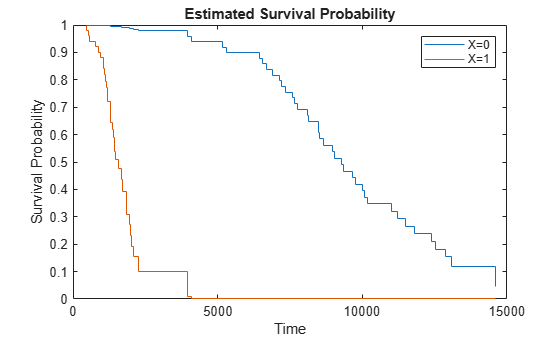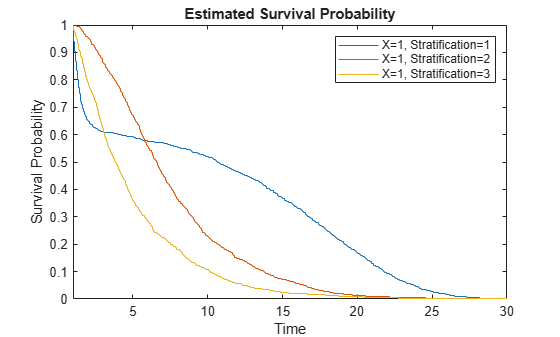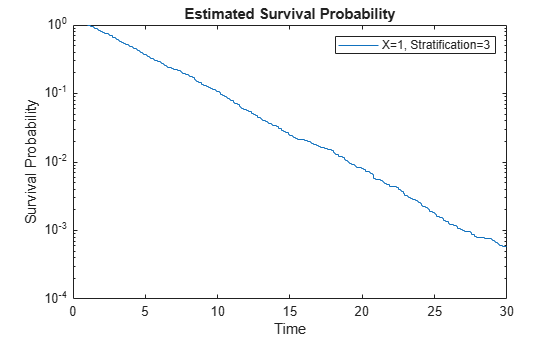# plotSurvival

Plot survival function of Cox proportional hazards model

## Syntax

``plotSurvival(coxMdl)``
``plotSurvival(coxMdl,X)``
``plotSurvival(coxMdl,X,Stratification)``
``plotSurvival(coxMdl,ax,___)``
``plotSurvival(___,Name,Value)``
``graphics = plotSurvival(___)``

## Description

example

````plotSurvival(coxMdl)` plots the baseline survival function of a Cox proportional hazards model `coxMdl`. The survival function at time `t` is the estimated probability of survival until time `t`. The term baseline refers to the survival function at the determined baseline of the predictors. This value is stored in `coxMdl.Baseline`, and the default value is the mean of the data set used for training.```

example

````plotSurvival(coxMdl,X)` plots the survival function when the predictors have the values in `X`. The plot includes one line for each row of `X`.```

example

````plotSurvival(coxMdl,X,Stratification)` plots the survival function for the given value of the stratification variable `Stratification`. You must have one row in `Stratification` for each row in `X`. NoteWhen you train `coxMdl` using stratification variables and pass predictor variables `X`, `plotSurvival` also requires you to pass stratification variables. ```

example

````plotSurvival(coxMdl,ax,___)` plots in the specified graphics axes `ax` using any of the input argument combinations in the previous syntaxes.```

example

````plotSurvival(___,Name,Value)` specifies additional options using one or more name-value arguments. For example, `plotSurvival(CoxMdl,"Time",T)` plots the survival function at times `T`.```
````graphics = plotSurvival(___)` returns an array of `Stair` graphics objects. See Stair Properties.```

## Examples

collapse all

Perform a Cox proportional hazards regression on the `lightbulb` data set, which contains simulated lifetimes of light bulbs. The first column of the light bulb data contains the lifetime (in hours) of two different types of bulbs. The second column contains a binary variable indicating whether the bulb is fluorescent or incandescent; 0 indicates the bulb is fluorescent, and 1 indicates it is incandescent. The third column contains the censoring information, where 0 indicates the bulb was observed until failure, and 1 indicates the observation was censored.

Fit a Cox proportional hazards model for the lifetime of the light bulbs, accounting for censoring. The predictor variable is the type of bulb.

```load lightbulb coxMdl = fitcox(lightbulb(:,2),lightbulb(:,1), ... 'Censoring',lightbulb(:,3));```

Plot the baseline survival function as a function of time `t`, meaning the probability that a light bulb fails after time `t`. By default, the baseline is calculated for the mean of the predictor, which in this case is `mean(lightbulb(:,2))` = `0.5`.

`plotSurvival(coxMdl)`Plot the survival for fluorescent bulbs (predictor = 0) and incandescent bulbs (predictor = 1).

`plotSurvival(coxMdl,[0;1])`To calculate the survival without plotting, use `survival`.

Load the `coxModel` data. (This simulated data is generated in the example Cox Proportional Hazards Model Object.) The model named `coxMdl` has three stratification levels (1, 2, and 3) and a predictor `X` with three categorical values (1, 1/20, and 1/100).

`load coxModel`

Plot the survival for `X = 1` at the three stratification levels.

```c1 = categorical(1); X = [c1;c1;c1]; stratification = [1;2;3]; plotSurvival(coxMdl,X,stratification) xlim([1,30])```Load the `coxModel` data. (This simulated data is generated in the example Cox Proportional Hazards Model Object.) The model named `coxMdl` has three stratification levels (1, 2, and 3) and a predictor `X` with three categorical values (1, 1/20, and 1/100).

`load coxModel`

To enable programmatic editing of a survival plot, create an axes.

```h = figure; axes1 = axes('Parent',h);```

Plot the survival function for the `X` predictor value `categorical(1)` and stratification level 3. This stratification level represents a constant hazard rate. When log-scaled, the resulting survival plot should, therefore, be close to a straight line. Plot for times 1 through 30.

```oo = categorical(1); plotSurvival(coxMdl,axes1,oo,3,'Time',linspace(1,30,300)); axes1.YScale = 'log';```## Input Arguments

collapse all

Fitted Cox proportional hazards model, specified as a `CoxModel` object. Create `coxMdl` using `fitcox`.

Predictors for the model, specified as an array of predictors of the same type used for training `coxMdl`. Each row of `X` represents one set of predictors.

Data Types: `double` | `table` | `categorical`

Stratification level, specified as a variable or variables of the same type used for training `coxMdl`. Specify the same number of rows in `Stratification` as in `X`.

Data Types: `single` | `double` | `logical` | `char` | `string` | `table` | `cell` | `categorical`

Axes for plotting, specified as a graphics axes object.

### Name-Value Arguments

Specify optional pairs of arguments as `Name1=Value1,...,NameN=ValueN`, where `Name` is the argument name and `Value` is the corresponding value. Name-value arguments must appear after other arguments, but the order of the pairs does not matter.

Before R2021a, use commas to separate each name and value, and enclose `Name` in quotes.

Example: `plotSurvival(CoxMdl,Time=T)`

Extrapolation method to compute the survival for out-of-range times, specified as one of the listed values. A `CoxModel` object uses the cumulative baseline hazard, stored in `CoxModel.Hazard`, to compute the baseline survival function in the `survival` or `plotSurvival` functions. For times within the range (defined next), results are from linear interpolation of the baseline survival function.

For a nonstratified model, the range is `[T1,T2]`, where `T1` is (1 - `eps`) times the earliest training time, and `T2` is the latest training time. The `ExtrapolationMethod` for a time `T` gives the following result:

• `'nearest'` (default) — If `T` < `T1`, the result is for time `T1`. If `T` > `T2`, the result is for time `T2`.

• `'linear'` — The result is a linear extrapolation from the nearest time in the range. Extrapolated survival values are truncated to lie in `[0,1]`. In other words, if `val` is the returned survival value and `extrapval` is the linear extrapolation, then

`val = max(0, min(1,extrapval))`.

• `'next'` — If `T` < `T1`, the result is for time `T1`. If `T` > `T2`, the result is `NaN`.

• `'none'` — If `T` < `T1` or `T` > `T2`, the result is `NaN`.

• `'previous'` — If `T` < `T1`, the result is `NaN`. If `T` > `T2`, the result is for time `T2`.

For each stratum in a stratified model, define the time range exactly as for a nonstratified model, using the event times in that stratum. The extrapolated values of survival in each stratum use the `ExtrapolationMethod` applied to the stratum range.

Example: `'next'`

Data Types: `char` | `string`

Times for survival estimates, specified as a real vector. `plotSurvival` sorts the specified times and converts them to a column vector, if necessary. For an unstratified model and times in the range of `coxMdl.Hazard(:,1)`, the resulting values are linearly interpolated from times in the training data. For `Time` values outside the fitting data range, the survival is extrapolated using the extrapolation method specified in `ExtrapolationMethod`.

For stratified models, distinct time ranges for each stratum in `coxMdl.Hazard(:,1)` are separated by 0s in `coxMdl.Hazard(:,2)`. `plotSurvival` estimates the survival in each stratum using the same procedure as for an unstratified model.

Example: `0:40`

Data Types: `double`

## Version History

Introduced in R2021a